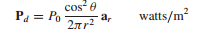### Create an Account

Already have account?

### Forgot Your Password ?

Home / Questions / Spherical surfaces at r 2 4 and 6 m carry uniform surface charge densities of 20 nCm2 4 n...

# Spherical surfaces at r 2 4 and 6 m carry uniform surface charge densities of 20 nCm2 4 nCm2 and ρS0 respectively a Find D at r 1 3 and 5 m b Determine ρS0 such that

Spherical surfaces at r = 2, 4, and 6 m carry uniform surface charge densities of 20 nC/m2, −4 nC/m2, and ρS0, respectively. (a) Find D at r = 1, 3, and 5 m. (b) Determine ρS0 such that D = 0 at r = 7 m

2. A certain light-emitting diode (LED) is centered at the origin with its surface in the xy plane. At far distances, the LED appears as a point, but the glowing surface geometry produces a far-field radiation pattern that follows a raised cosine law: that is, the optical power (flux) density in watts/m2 is given in spherical coordinates bywhere θ is the angle measured with respect to the direction that is normal to the LED surface (in this case, the z axis), and r is the radial distance from the origin at which the power is detected. (a) In terms of P0, find the total power in watts emitted in the upper half-space by the LED; (b) Find the cone angle, θ1, within which half the total power is radiated, that is, within the range 0

Jun 14 2020 View more View Less

#### Answer (Solved)Subscribe To Get Solution